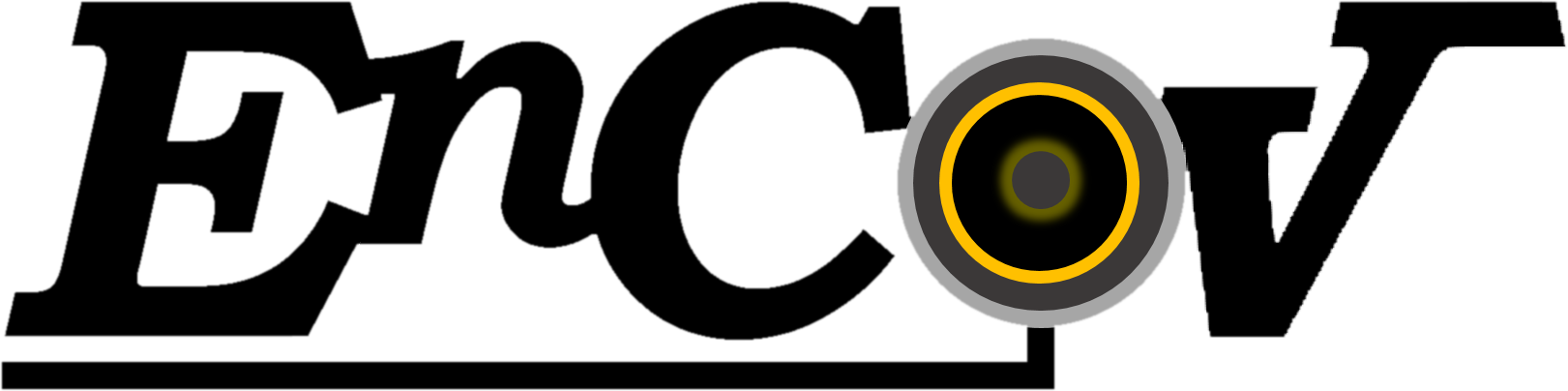# Endoscopy and Computer Vision

### EnCoV is a research group located at the Faculty of Medicine in Clermont-Ferrand. It has links with the local university and university hospital. It is part of the Image-Guided Therapies research axis of Institut Pascal (UMR6602 UCA, CNRS, INP Clermont Auvergne) and the Technology for Medicine (TechMed) research axis of CHU Clermont-Ferrand.

Back to index
We do research in computer vision, endoscopy and computer-aided endoscopy. Below is a list of subjects and open problems.

### Geometry

#### Find the point pairs aligned with some direction where the tangent slopes of two conics match

Imagine that we have two conics $$A$$ and $$B$$ represented by their $$3\times3$$ homogeneous symmetric matrices and a direction $$d$$ represented by a $$2\times1$$ homogeneous vector. The question we ask here is for which point pairs $$q,p$$, each represented by a $$3\times1$$ homogeneous vectors, on $$A,B$$ respectively and forming a line with direction $$d$$, do the slope of the tangents to $$A$$ and $$B$$ at $$p$$ and $$q$$ respectively match? An easier question to start is to determine how many such point pairs exist in the complex Euclidean plane and then in the real Euclidean plane.

Website created and maintained by Adrien Bartoli.